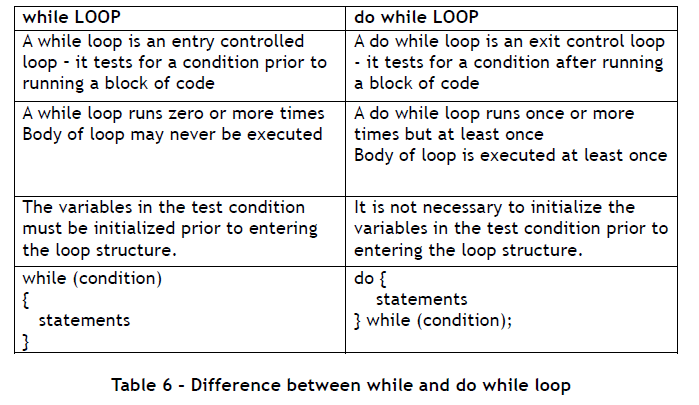Home » Online Computer Education » Learn Fundamentals of Java Programming “The do while statement in Java Programming ” Lesson 13

# Learn Fundamentals of Java Programming “The do while statement in Java Programming ” Lesson 13

The do while statement

The do while statement evaluates the test after executing the body of a loop. The structure of the Java do-while statement is as below:

do
{
statements
} while (expression);

Let us write the same program to print the squares of numbers from 1 to 5. This time we will use a do-while loop. The following steps need to be performed.

1. Initialize number = 1
2. Print the square of the number
3. Increment number (number = number + 1)
4. Test if the number <= 5
5. If yes, Go back to step 2
If no, Exit the loop

The tasks that need to be performed repeatedly are in steps 2 and 3 – these constitute the body of the loop. The test condition is in step 4. The corresponding Java code is as below:

public class DoWhileDemo {
public static void main(String[] args) {
int number = 1;
do{
System.out.print(“Square of ” + number);
System.out.println(” = ” + number*number);
++ number;
} while (number <= 5);
}
}

The output of the DoWhileDemo program is the same as Figure 1.4f. You might be wondering if both the while and do-while loops give the same output, what is the use of having two types of loop constructs. Well, consider the case when the variable number is initialized to 6.

int number = 6;

The while loop would first test whether number <= 5. Since 6 > 5, the condition is false and the loop body would not be entered and as a result nothing is displayed in the output window. However in the case of the do-while loop, since the body of the loop is executed before the test condition, the square of 6 is printed (“Square of 6 = 36”), then, number is incremented to 7. Now the test for number >= 5 is made. Since 7 > 5, the condition is false and the loop is exited. To summarize, the do while loop always executes one or more times whereas the while loop executes zero or more times.
Table 6 summarizes the difference between a while and a do-while loop.Common Coding Errors: Loops

1. Infinite Loops
This type of error occurs when the loop runs forever, it is never exited. This happens when the test condition is always true.

For example,

int number = 1 ;
while (number <= 5)
{
System.out.print(“Square of ” + number);
System.out.println(” = ” + number*number);
}

This loop will run forever since number never changes – it remains at 1.
2. Syntax Errors

1. Do not forget the semi colon at the end of the test condition in a do-while loop. Otherwise you will get a compiler error.

2. Do not place a semi colon at the end of the test condition in a while loop.
For example,

int x = 1;
while( x <=5 );
x = x +1;

This loop is an infinite loop – The semicolon after the while causes the statement to be repeated as the null statement (which does nothing). If the semi colon at the end is be removed, the loop will work as expected.The main objective of this website is to provide quality study material to all students (from 1st to 12th class of any board) irrespective of their background as our motto is “Education for Everyone”. It is also a very good platform for teachers who want to share their valuable knowledge.